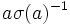# Commutator of element and automorphism

## Definition

### Basic definition

Let$G$ be a group,$a \in G$ be an element, and$\sigma \in \operatorname{Aut}(G)$ be an automorphism of$G$. Then, the commutator of$a$ and$\sigma$, denoted$[a,\sigma]$ is sometimes defined as (for those following the left-action convention):$a\sigma(a)^{-1}$

Under the right-action convention, the commutator is written as$[\sigma,a]$ and is defined as:$\sigma(a)^{-1}a$

The notion of commutator gives the usual notion of commutator of two elements$a$ and$b$, if we take$\sigma$ as conjugation by$b$ (left and right notions respectively).

### Definition as an ordinary commutator of elements

Let$G$ be a group,$a \in G$ be an element and$\sigma \in \operatorname{Aut}(G)$. Consider the external semidirect product$G \rtimes \langle \sigma \rangle$ (we can take the semidirect product of$G$ with any subgroup of$\operatorname{Aut}(G)$ containing$\sigma$). The commutator of$a$ and$\sigma$ is simply the commutator of these as elements in the semidirect product.